# CLASS 9 MATHS CHAPTER-8 QUADRILATERALS

### Exercise 8.1

Question 1. The angles of quadrilateral are in the ratio 3 : 5 : 9 : 13. Find all the angles of the quadrilateral.

Solution:
Let the angles of the quadrilateral be 3x, 5x, 9x and 13x.
∴ 3x + 5x + 9x + 13x = 360°
[Angle sum property of a quadrilateral]
⇒ 30x = 360°
⇒ x =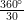= 12°
∴ 3x = 3 x 12° = 36°
5x = 5 x 12° = 60°
9x = 9 x 12° = 108°
13a = 13 x 12° = 156°
⇒ The required angles of the quadrilateral are 36°, 60°, 108° and 156°.

Question 2. If the diagonals of a parallelogram are equal, then show that it is a rectangle.
Solution:
Let ABCD is a parallelogram such that AC = BD.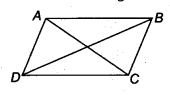In ∆ABC and ∆DCB,
AC = DB [Given]
AB = DC [Opposite sides of a parallelogram]
BC = CB [Common]
∴ ∆ABC ≅ ∆DCB [By SSS congruency]
⇒ ∠ABC = ∠DCB [By C.P.C.T.] …(1)
Now, AB || DC and BC is a transversal. [ ∵ ABCD is a parallelogram]
∴ ∠ABC + ∠DCB = 180° … (2) [Co-interior angles]
From (1) and (2), we have
∠ABC = ∠DCB = 90°
i.e., ABCD is a parallelogram having an angle equal to 90°.
∴ ABCD is a rectangle.

Question 3. Show that if the diagonals of a quadrilateral bisect each other at right angles, then it is a rhombus.
Solution:
Let ABCD be a quadrilateral such that the diagonals AC and BD bisect each other at right angles at O.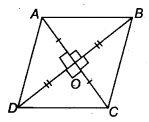∴ In ∆AOB and ∆AOD, we have
AO = AO [Common]
OB = OD [O is the mid-point of BD]
∠AOB = ∠AOD [Each 90]
∴ ∆AQB ≅ ∆AOD [By,SAS congruency
∴ AB = AD [By C.P.C.T.] ……..(1)
Similarly, AB = BC .. .(2)
BC = CD …..(3)
CD = DA ……(4)
∴ From (1), (2), (3) and (4), we have
AB = BC = CD = DA
Thus, the quadrilateral ABCD is a rhombus.
Alternatively : ABCD can be proved first a parallelogram then proving one pair of adjacent sides equal will result in rhombus.

Question 4. Show that the diagonals of a square are equal and bisect each other at right angles.
Solution:
Let ABCD be a square such that its diagonals AC and BD intersect at O.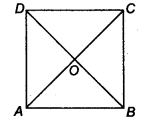(i) To prove that the diagonals are equal, we need to prove AC = BD.
In ∆ABC and ∆BAD, we have
AB = BA [Common]
BC = AD [Sides of a square ABCD]
∠ABC = ∠BAD [Each angle is 90°]
∴ ∆ABC ≅ ∆BAD [By SAS congruency]
AC = BD [By C.P.C.T.] …(1)

(ii) AD || BC and AC is a transversal. [∵ A square is a parallelogram]
∴ ∠1 = ∠3
[Alternate interior angles are equal]
Similarly, ∠2 = ∠4
Now, in ∆OAD and ∆OCB, we have
AD = CB [Sides of a square ABCD]
∠1 = ∠3 [Proved]
∠2 = ∠4 [Proved]
∴ ∆OAD ≅ ∆OCB [By ASA congruency]
⇒ OA = OC and OD = OB [By C.P.C.T.]
i.e., the diagonals AC and BD bisect each other at O. …….(2)

(iii) In ∆OBA and ∆ODA, we have
OB = OD [Proved]
BA = DA [Sides of a square ABCD]
OA = OA [Common]
∴ ∆OBA ≅ ∆ODA [By SSS congruency]
⇒ ∠AOB = ∠AOD [By C.P.C.T.] …(3)
∵ ∠AOB and ∠AOD form a linear pair.
∴∠AOB + ∠AOD = 180°
∴∠AOB = ∠AOD = 90° [By(3)]
⇒ AC ⊥ BD …(4)
From (1), (2) and (4), we get AC and BD are equal and bisect each other at right angles.

Question 5. Show that if the diagonals of a quadrilateral are equal and bisect each other at right angles, then it is a square.
Solution:
Let ABCD be a quadrilateral such that diagonals AC and BD are equal and bisect each other at right angles.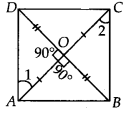Now, in ∆AOD and ∆AOB, We have
∠AOD = ∠AOB [Each 90°]
AO = AO [Common]
OD = OB [ ∵ O is the midpoint of BD]
∴ ∆AOD ≅ ∆AOB [By SAS congruency]
⇒ AD = AB [By C.P.C.T.] …(1)
Similarly, we have
AB = BC … (2)
BC = CD …(3)
CD = DA …(4)
From (1), (2), (3) and (4), we have
AB = BC = CD = DA
∴ Quadrilateral ABCD have all sides equal.
In ∆AOD and ∆COB, we have
AO = CO [Given]
OD = OB [Given]
∠AOD = ∠COB [Vertically opposite angles]
So, ∆AOD ≅ ∆COB [By SAS congruency]
∴∠1 = ∠2 [By C.P.C.T.]
But, they form a pair of alternate interior angles.
Similarly, AB || DC
∴ ABCD is a parallelogram.
∴ Parallelogram having all its sides equal is a rhombus.
∴ ABCD is a rhombus.
Now, in ∆ABC and ∆BAD, we have
AC = BD [Given]
AB = BA [Common]
∴ ∆ABC ≅ ∆BAD [By SSS congruency]
∴ ∠ABC = ∠BAD [By C.P.C.T.] ……(5)
Since, AD || BC and AB is a transversal.
∴∠ABC + ∠BAD = 180° .. .(6) [ Co – interior angles]
⇒ ∠ABC = ∠BAD = 90° [By(5) & (6)]
So, rhombus ABCD is having one angle equal to 90°.
Thus, ABCD is a square.

Question 6. Diagonal AC of a parallelogram ABCD bisects ∠A (see figure). Show that
(i) it bisects ∠C also,
(ii) ABCD is a rhombus.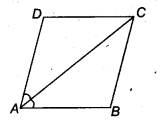Solution:
We have a parallelogram ABCD in which diagonal AC bisects ∠A
⇒ ∠DAC = ∠BAC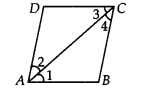(i) Since, ABCD is a parallelogram.
∴ AB || DC and AC is a transversal.
∴ ∠1 = ∠3 …(1)
[ ∵ Alternate interior angles are equal]
Also, BC || AD and AC is a transversal.
∴ ∠2 = ∠4 …(2)
[ v Alternate interior angles are equal]
Also, ∠1 = ∠2 …(3)
[ ∵ AC bisects ∠A]
From (1), (2) and (3), we have
∠3 = ∠4
⇒ AC bisects ∠C.

(ii) In ∆ABC, we have
∠1 = ∠4 [From (2) and (3)]
⇒ BC = AB …(4)
[ ∵ Sides opposite to equal angles of a ∆ are equal]
But, ABCD is a parallelogram. [Given]
∴ AB = DC …(6)
From (4), (5) and (6), we have
AB = BC = CD = DA
Thus, ABCD is a rhombus.

Question 7. ABCD is a rhombus. Show that diagonal AC bisects ∠Aas well as ∠C and diagonal BD bisects ∠B as well AS ∠D.
Solution:
Since, ABCD is a rhombus.
⇒ AB = BC = CD = DA
Also, AB || CD and AD || BC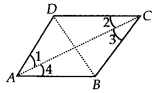Now, CD = AD ⇒ ∠1 = ∠2 …….(1)
[ ∵ Angles opposite to equal sides of a triangle are equal]
Also, AD || BC and AC is the transversal.
[ ∵ Every rhombus is a parallelogram]
⇒ ∠1 = ∠3 …(2)
[ ∵ Alternate interior angles are equal]
From (1) and (2), we have
∠2 = ∠3 …(3)
Since, AB || DC and AC is transversal.
∴ ∠2 = ∠4 …(4)
[ ∵ Alternate interior angles are equal] From (1) and (4),
we have ∠1 = ∠4
∴ AC bisects ∠C as well as ∠A.
Similarly, we can prove that BD bisects ∠B as well as ∠D.

Question 8. ABCD is a rectangle in which diagonal AC bisects ∠A as well as ∠C. Show that
(i) ABCD is a square
(ii) diagonal BD bisects ∠B as well as ∠D.

Solution:
We have a rectangle ABCD such that AC bisects ∠A as well as ∠C.
i.e., ∠1 = ∠4 and ∠2 = ∠3 ……..(1)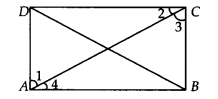(i) Since, every rectangle is a parallelogram.
∴ ABCD is a parallelogram.
⇒ AB || CD and AC is a transversal.
∴∠2 = ∠4 …(2)
[ ∵ Alternate interior angles are equal]
From (1) and (2), we have
∠3 = ∠4
In ∆ABC, ∠3 = ∠4
⇒ AB = BC
[ ∵ Sides opposite to equal angles of a A are equal]
Similarly, CD = DA
So, ABCD is a rectangle having adjacent sides equal.
⇒ ABCD is a square.

(ii) Since, ABCD is a square and diagonals of a square bisect the opposite angles.
So, BD bisects ∠B as well as ∠D.

Question 9. In parallelogram ABCD, two points P and Q are taken on diagonal BD such that DP = BQ (see figure). Show that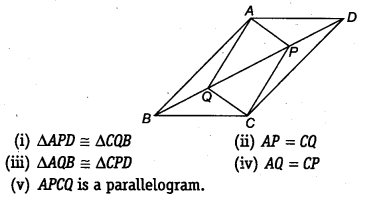Solution:
We have a parallelogram ABCD, BD is the diagonal and points P and Q are such that PD = QB

(i) Since, AD || BC and BD is a transversal.
∴ ∠ADB = ∠CBD [ ∵ Alternate interior angles are equal]
Now, in ∆APD and ∆CQB, we have
AD = CB [Opposite sides of a parallelogram ABCD are equal]
PD = QB [Given]
∴ ∆APD ≅ ∆CQB [By SAS congruency]

(ii) Since, ∆APD ≅ ∆CQB [Proved]
⇒ AP = CQ [By C.P.C.T.]

(iii) Since, AB || CD and BD is a transversal.
∴ ∠ABD = ∠CDB
⇒ ∠ABQ = ∠CDP
Now, in ∆AQB and ∆CPD, we have
QB = PD [Given]
∠ABQ = ∠CDP [Proved]
AB = CD [ Y Opposite sides of a parallelogram ABCD are equal]
∴ ∆AQB = ∆CPD [By SAS congruency]

(iv) Since, ∆AQB = ∆CPD [Proved]
⇒ AQ = CP [By C.P.C.T.]

Opposite sides are equal. [Proved]
∴ ∆PCQ is a parallelogram.

Question 10. ABCD is a parallelogram and AP and CQ are perpendiculars from vertices A and C on diagonal BD (see figure). Show that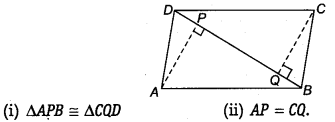Solution:
(i) In ∆APB and ∆CQD, we have
∠APB = ∠CQD [Each 90°]
AB = CD [ ∵ Opposite sides of a parallelogram ABCD are equal]
∠ABP = ∠CDQ
[ ∵ Alternate angles are equal as AB || CD and BD is a transversal]
∴ ∆APB = ∆CQD [By AAS congruency]

(ii) Since, ∆APB ≅ ∆CQD [Proved]
⇒ AP = CQ [By C.P.C.T.]

Question 11. In ∆ABC and ∆DEF, AB = DE, AB || DE, BC – EF and BC || EF. Vertices A, B and C are joined to vertices D, E and F, respectively (see figure).
Show that
(i) quadrilateral ABED is a parallelogram
(ii) quadrilateral BEFC is a parallelogram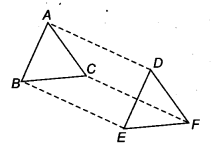(iv) quadrilateral ACFD is a parallelogram
(v) AC = DF
(vi) ∆ABC ≅ ∆DEF

Solution:
(i) We have AB = DE [Given]
and AB || DE [Given]
i. e., ABED is a quadrilateral in which a pair of opposite sides (AB and DE) are parallel and of equal length.
∴ ABED is a parallelogram.

(ii) BC = EF [Given]
and BC || EF [Given]
i.e. BEFC is a quadrilateral in which a pair of opposite sides (BC and EF) are parallel and of equal length.
∴ BEFC is a parallelogram.

(iii) ABED is a parallelogram [Proved]
[ ∵ Opposite sides of a parallelogram are equal and parallel] Also, BEFC is a parallelogram. [Proved]
BE || CF and BE = CF …(2)
[ ∵ Opposite sides of a parallelogram are equal and parallel]
From (1) and (2), we have

i.e., In quadrilateral ACFD, one pair of opposite sides (AD and CF) are parallel and of equal length.

(v) Since, ACFD is a parallelogram. [Proved]
So, AC =DF [∵ Opposite sides of a parallelogram are equal]

(vi) In ∆ABC and ∆DFF, we have
AB = DE [Given]
BC = EF [Given]
AC = DE [Proved in (v) part]
∆ABC ≅ ∆DFF [By SSS congruency]

Question 12. ABCD is a trapezium in which AB || CD and AD = BC (see figure). Show that
(i )∠A=∠B
(ii )∠C=∠D
(iv) diagonal AC = diagonal BD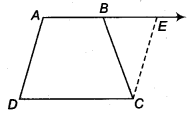[Hint: Extend AB and draw a line through C parallel to DA intersecting AB produced at E].

Solution:
We have given a trapezium ABCD in which AB || CD and AD = BC.

(i) Produce AB to E and draw CF || AD.. .(1)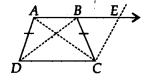∵ AB || DC
⇒ AE || DC Also AD || CF
∴ AECD is a parallelogram.
[ ∵ Opposite sides of the parallelogram are equal]
But AD = BC …(2) [Given]
By (1) and (2), BC = CF
Now, in ∆BCF, we have BC = CF
⇒ ∠CEB = ∠CBE …(3)
[∵ Angles opposite to equal sides of a triangle are equal]
Also, ∠ABC + ∠CBE = 180° … (4)
[Linear pair]
and ∠A + ∠CEB = 180° …(5)
[Co-interior angles of a parallelogram ADCE]
From (4) and (5), we get
∠ABC + ∠CBE = ∠A + ∠CEB
⇒ ∠ABC = ∠A [From (3)]
⇒ ∠B = ∠A …(6)

(ii) AB || CD and AD is a transversal.
∴ ∠A + ∠D = 180° …(7) [Co-interior angles]
Similarly, ∠B + ∠C = 180° … (8)
From (7) and (8), we get
∠A + ∠D = ∠B + ∠C
⇒ ∠C = ∠D [From (6)]

(iii) In ∆ABC and ∆BAD, we have
AB = BA [Common]
∴ ∆ABC = ∆BAD [By SAS congruency]

(iv) Since, ∆ABC = ∆BAD [Proved]
⇒ AC = BD [By C.P.C.T.]

### NCERT Solutions for Class 9 Maths Exercise 8.2

Question 1. ABCD is a quadrilateral in which P, Q, R and S are the mid-points of sides AB, BC, CD and DA respectively (See figure). AC is a diagonal. Show that :

(i) SR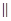AC and SR =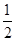AC

(ii) PQ = SR

(iii) PQRS is a parallelogram.

Solution:
In ABC, P is the mid-point of AB and Q is the mid-point of BC.

Then PQAC and PQ =AC

(i) In ACD, R is the mid-point of CD and S is the mid-point of AD.

Then SRAC and SR =AC

(ii) Since PQ =AC and SR =AC

Therefore, PQ = SR

(iii) Since PQAC and SRAC

Therefore, PQSR [two lines parallel to given line are parallel to each other]

Now PQ = SR and PQSR

Therefore, PQRS is a parallelogram.

Question 2. ABCD is a rhombus and P, Q, R, S are mid-points of AB, BC, CD and DA respectively. Prove that quadrilateral PQRS is a rectangle.

Solution:
Given: P, Q, R and S are the mid-points of respective sides AB, BC, CD and DA of rhombus. PQ, QR, RS and SP are joined.

To prove: PQRS is a rectangle.

Construction: Join A and C.

Proof: In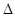ABC, P is the mid-point of AB and Q is the mid-point of BC.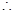PQAC and PQ =AC ……….(i)

InADC, R is the mid-point of CD and S is the mid-point of AD.SRAC and SR =AC ……….(ii)

From eq. (i) and (ii), PQSR and PQ = SRPQRS is a parallelogram.

Now ABCD is a rhombus. [Given]AB = BC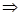AB =BCPB = BQ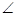1 =2 [Angles opposite to equal sides are equal]

Now in triangles APS and CQR, we have,

AP = CQ [P and Q are the mid-points of AB and BC and AB = BC]

Similarly, AS = CR and PS = QR [Opposite sides of a parallelogram]APS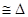CQR [By SSS congreuancy]3 =4 [By C.P.C.T.]

Now we have1 +SPQ +3 =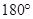And2 +PQR +4 =[Linear pairs]1 +SPQ +3 =2 +PQR +4

Since1 =2 and3 =4 [Proved above]SPQ =PQR ……….(iii)

Now PQRS is a parallelogram [Proved above]SPQ +PQR =……….(iv) [Interior angles]

Using eq. (iii) and (iv),SPQ +SPQ =2SPQ =SPQ =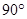Hence PQRS is a rectangle.

Question 3. ABCD is a rectangle and P, Q, R and S are the mid-points of the sides AB, BC, CD and DA respectively. Show that the quadrilateral PQRS is a rhombus.

Solution:
Given: A rectangle ABCD in which P, Q, R and S are the mid-points of the sides AB, BC, CD and DA respectively. PQ, QR, RS and SP are joined.

To prove: PQRS is a rhombus.

Construction: Join AC.

Proof: In ABC, P and Q are the mid-points of sides AB, BC respectively.

PQ AC and PQ = AC ……….(i)

In ADC, R and S are the mid-points of sides CD, AD respectively.

SR AC and SR = AC ……….(ii)

From eq. (i) and (ii), PQ SR and PQ = SR ……….(iii)

PQRS is a parallelogram.

Now ABCD is a rectangle. [Given]

AD = BC AS = BQ ……….(iv)

In triangles APS and BPQ,

AP = BP [P is the mid-point of AB]

PAS = PBQ [Each ]

And AS = BQ [From eq. (iv)]

APS BPQ [By SAS congruency]

PS = PQ [By C.P.C.T.] ………(v)

From eq. (iii) and (v), we get that PQRS is a parallelogram.

PS = PQ

Hence, PQRS is a rhombus.

Question 4. ABCD is a trapezium, in which AB DC, BD is a diagonal and E is the mid-point of AD. A line is drawn through E, parallel to AB intersecting BC at F (See figure). Show that F is the mid-point of BC.

Solution:
Let diagonal BD intersect line EF at point P.

In DAB,

E is the mid-point of AD and EP AB [ EF AB (given) P is the part of EF]

P is the mid-point of other side, BD of DAB.

[A line drawn through the mid-point of one side of a triangle, parallel to another side intersects the third side at the mid-point]

Now in BCD,

P is the mid-point of BD and PF DC [EF AB (given) and AB DC (given)]

EF DC and PF is a part of EF.

F is the mid-point of other side, BC of BCD. [Converse of mid-point of theorem]

Question 5. In a parallelogram ABCD, E and F are the mid-points of sides AB and CD respectively (See figure). Show that the line segments AF and EC trisect the diagonal BD.

Solution:
Since E and F are the mid-points of AB and CD respectively.

AE = AB and CF = CD……….(i)

But ABCD is a parallelogram.

AB = CD and AB DC

AB = CD and AB DC

AE = FC and AE FC [From eq. (i)]

AECF is a parallelogram.

FA CE FP CQ [FP is a part of FA and CQ is a part of CE] ………(ii)

Since the segment drawn through the mid-point of one side of a triangle and parallel to the other side bisects the third side.

In DCQ, F is the mid-point of CD and FP CQ

P is the mid-point of DQ.

DP = PQ ……….(iii)

Similarly, In ABP, E is the mid-point of AB and EQ AP

Q is the mid-point of BP.

BQ = PQ ……….(iv)

From eq. (iii) and (iv),

DP = PQ = BQ ………(v)

Now BD = BQ + PQ + DP = BQ + BQ + BQ = 3BQ

BQ = BD ……….(vi)

From eq. (v) and (vi),

DP = PQ = BQ = BD

Points P and Q trisects BD.

So AF and CE trisects BD.

Question 6. Show that the line segments joining the mid-points of opposite sides of a quadrilateral bisect each other.

Solution:
Given: A quadrilateral ABCD in which EG and FH are the line-segments joining the mid-points of opposite sides of a quadrilateral.

To prove: EG and FH bisect each other.

Construction: Join AC, EF, FG, GH and HE.

Proof: In ABC, E and F are the mid-points of respective sides AB and BC.

EF AC and EF AC ……….(i)

G and H are the mid-points of respective sides CD and AD.

HG AC and HG AC ……….(ii)

From eq. (i) and (ii),

EF HG and EF = HG

EFGH is a parallelogram.

Since the diagonals of a parallelogram bisect each other, therefore line segments (i.e. diagonals) EG and FH (of parallelogram EFGH) bisect each other.

Question 7. ABC is a triangle right angled at C. A line through the mid-point M of hypotenuse AB and parallel to BC intersects AC at D.

Solution:
(i) In ABC, M is the mid-point of AB [Given]

MD BC

AD = DC [Converse of mid-point theorem]

Thus D is the mid-point of AC.

(ii) BC (given) consider AC as a transversal.

1 = C [Corresponding angles]

1 = [C = ]

Thus MD AC.

(iii) In AMD and CMD,

1 = 2 = [proved above]

MD = MD [common]

AMD CMD [By SAS congruency]

AM = CM [By C.P.C.T.] ……….(i)

Given that M is the mid-point of AB.

AM = AB ……….(ii)

From eq. (i) and (ii),

CM = AM = AB

Get 30% off your first purchase!

X
error: Content is protected !!
Scroll to Top